# Plasma reforming of toluene as a model tar compound from biomass gasification: effect of CO2 and steam

## Abstract

In this study, plasma reforming of toluene as a tar model compound from biomass gasification has been carried out using an AC gliding arc discharge reactor. The influence of steam and CO2 addition on the reforming of toluene has been evaluated. The results show that the highest toluene conversion (59.9%) was achieved when adding 3 vol% CO2 at a toluene concentration of 16.1 g/Nm3 and a specific energy input of 0.25 kWh/m3. Further increasing CO2 concentration to 12 vol% decreased the conversion of toluene. The presence of steam in the plasma CO2 reforming of toluene creates oxidative OH radicals which contribute to the enhanced conversion of toluene and energy efficiency of the plasma reforming process through stepwise oxidation of toluene and reaction intermediates. Hydrogen and C2H2 were identified as the major gas products in the plasma reforming of toluene without CO2 or steam, with a yield of 9.7% and 14.5%, respectively, while syngas was the primary products with a maximum yield of 58.3% (27.5% for H2 and 30.8% for CO) in the plasma reforming with the addition of 12 vol% CO2. The plausible reaction pathways and mechanism in the plasma reforming of toluene have been proposed through the combination of the analysis of gas and condensed products and spectroscopic diagnostics.

## Introduction

One of the major challenges in the gasification of biomass waste is the contamination of producer gas with tar, an undesirable by-product, consisting of mixed condensable aromatics . The presence of tar in the producer gas causes the safety issues for the operation of biomass gasifiers and downstream equipment and decreases the recoverable calorific value from biomass. Specifically, tar condensation can occur in pipelines and turbine engines at high concentration levels, resulting in blockage in the intake manifold and fouling effects on turbine blades [1, 2]. The concentration of tar in producer gas is in the range of 1–100 g/Nm3, depending on the processing conditions and the type of gasifier, while the acceptable level of tar in the downstream facilities should be less than 100 mg/Nm3 [3, 4]. In addition, tar contains carcinogenic compounds, which can be deposited in the respiratory tract and even can damage the central nervous system at high concentrations, with a subsequent increase of the potential health effects .

To eliminate and transform tar in fuel gas, great efforts have been dedicated to investigating different technologies for tar removal, including mechanical separation, such as sorbent or surfactant absorption , thermal cracking , and catalytic oxidation [1, 8]. The physical separation process is efficient for the removal of tars with a low concentration and simple structure. However, this process might not work if the concentration and number of rings of aromatic molecules increase. Significant tar removal can be achieved using thermal cracking at higher temperatures (over 1200 °C) at the expense of higher energy consumption and cost . Catalytic reforming is a very attractive process, since tar can be converted into syngas, an important feedstock for the synthesis of a range of chemicals and fuels. However, rapid deactivation of the catalysts resulted from catalyst agglomeration, poisoning, and coke deposition remains a critical challenge for this process to be used on a commercial scale due to the complex composition of tars and relatively high operation temperatures (over 600 °C) .

Non-thermal plasma (NTP) technology has been considered as a promising and emerging alternative to thermal and catalytic processes for the conversion of tars at low temperatures and ambient pressure [10,11,12]. Highly energetic electrons generated in NTP collide with background gases or reactants, creating a cascade of reactive species including radicals, excited atoms, molecules, and ions, to activate a range of chemical reactions [13,14,15]. Note that some of these species are not available in chemical reactions using the conventional thermal or catalytic processes, and thus, new reaction routes can be initiated using NTP technology. In the recent years, different NTP systems have been developed for the removal of tars, including corona discharge [16,17,18], dielectric barrier discharge [19,20,21,22], microwave discharge [11, 23], spark discharge , and gliding arc discharge (GAD) [25,26,27,28,29]. Compared to other NTP systems, GAD shows higher energy efficiency for gas conversion due to its significantly higher electron density (1023–1024 m−3) and higher flexibility of working in a wide range of flow rates and elevated power levels efficiently .

Most of the previous works focused on the conversion of a single tar model compound or mixed tar compounds using NTP. However, the producer gas is usually a mixture of syngas, CO2, steam, and hydrocarbons. For instance, the concentration of CO2 in the producer gas is around 10–13 vol%, while H2O accounts for about 10 vol% . Thus, it is important to understand how the presence of CO2 and steam affects plasma reforming of tars and the role of CO2 and steam in the creation of new reaction pathways contributed to the conversion of tars, which is critical for the further optimization of plasma tar reforming processes.

In this study, an AC gliding arc discharge reactor has been developed for the reforming of toluene as a tar model compound. The effect of CO2 addition and steam-to-carbon (S/C) molar ratio on the performance of plasma reforming of toluene has been evaluated in terms of the conversion of toluene, the yield of gaseous products, and the energy efficiency of the process. Optical emission spectroscopic (OES) diagnostics have been used to investigate the formation of reactive species generated in the plasma reforming process. The plausible reaction mechanisms and pathways involved in the plasma toluene reforming process have been elucidated using OES diagnostics combined with a comprehensive analysis of gas and liquid products.

## Experimental

### Experimental setup

Figure 1 shows the schematic diagram of the experimental setup. A lab-scale GAD reactor with two knife-shaped stainless-steel electrodes (60 mm long and 18 mm wide) was used in this experiment. The electrodes were fixed on a Teflon plate and symmetrically placed on both sides of a gas nozzle with a diameter of 1.5 mm. The narrowest gap between the electrodes was 2 mm for the initial arc formation (electrode throat) and the vertical distance between the nozzle outlet and the electrode throat was 3 mm. Nitrogen was used as a carrier gas. To understand the effect of CO2 on tar reforming, a mixture of nitrogen and CO2 was also used in the experiment. A mixed stream (toluene, deionized water and carrier gas) was preheated to 200 °C in a tube furnace for generating a steady-state vapor before injecting into the GAD reactor. The flow of toluene and deionized water can be controlled by high-resolution syringe pumps (KDS Legato 100). To understand the effect of steam on tar reforming, the steam-to-carbon ratio was varied from 0 to 2.5. The total flow rate of the gas mixture was fixed at 3.5 L/min with a constant toluene concentration of 16.1 g/Nm3 in the experiment.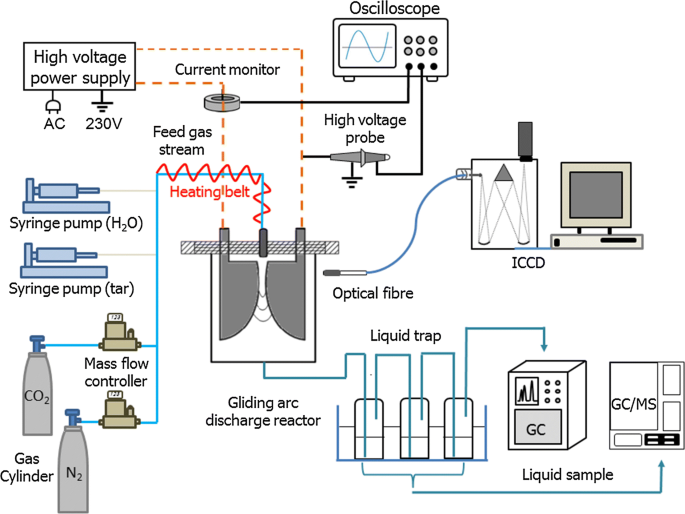Fig. 1

The GAD reactor was powered by a neon transformer with a maximum peak-to-peak voltage of 10 kV and a frequency of 50 Hz. A high voltage probe (Testec, TT-HVP 15 HF) was used to measure the arc voltage of the GAD, while a current monitor (Magnelab, CT-E 0.5-BNC) was employed to measure the arc current. All the electrical signals were recorded by a four-channel digital oscilloscope (Tektronix, MDO 3024).

### Method of analysis and the definition of parameters

Gaseous products were analyzed by gas chromatography (Shimadzu, GC-2014) equipped with a thermal conductivity detector (TCD) and a flame ionization detector (FID). An ice-cold trap including three successive absorption bottles was placed at the exit of the GAD reactor to collect the condensable products in the effluent. The first two bottles contained dichloromethane (DCM) to absorb condensable products, while the last one was kept empty to collect remaining entrained droplets. The condensed liquid products were analyzed by gas chromatography/mass spectrometry (GC/MS Agilent 7820A MSD 5975C) and qualitatively identified using a mass spectral library from the National Institutes for Standards and Technology (NIST). All the measurements were performed and repeated three times after running the plasma reaction for about 40 min when a steady state was reached. Optical emission spectroscopic diagnostics of the GAD plasma was carried out using a Princeton Instruments spectrometer (Model 320 PI) with a focal length of 320 mm. An optical fiber was used to record the emission spectra of the GAD under different experimental conditions.

The steam-to-carbon molar ratio is defined as follows:

$${\raise0.7ex\hbox{S} \!\mathord{\left/ {\vphantom {S C}}\right.\kern-0pt} \!\lower0.7ex\hbox{C}} = \frac{{{\text{moles}}\;{\text{of}}\;{\text{H}}_{ 2} {\text{O}}}}{{7 \times {\text{moles}}\;{\text{of}}\;{\text{C}}_{ 7} {\text{H}}_{ 8} }}.$$
(1)

The discharge power (P) is calculated by the integration of arc voltage U and arc current I, as shown in the following equation:

$$P\left( W \right) = \frac{1}{T}\int\limits_{0}^{t = T} {U\left( t \right)} \times I\left( t \right){\text{d}}t.$$
(2)

The specific energy input (SEI) is defined as follows:

$${\text{SEI}}\left( {{{\text{kWh}} \mathord{\left/ {\vphantom {{\text{kWh}} {{\text{m}}^{ 3} }}} \right. \kern-0pt} {{\text{m}}^{ 3} }}} \right) = \frac{{P\left( {\text{kW}} \right)}}{{{\text{Total}}\;{\text{gas}}\;{\text{flow}}\;{\text{rate}}\;\left( {{{{\text{m}}^{3} } \mathord{\left/ {\vphantom {{{\text{m}}^{3} } {\text{h}}}} \right. \kern-0pt} {\text{h}}}} \right)}}.$$
(3)

The conversion (X) of toluene and the yield (Y) of major gaseous products is expressed as follows:

$$X_{{{\text{C}}_{7} {\text{H}}_{8} }} \left( \% \right) = \frac{{{\text{moles}}\;{\text{of}}\;{\text{toluene}}\;{\text{input}} - {\text{moles}}\;{\text{of}}\;{\text{toluene}}\;{\text{effluent }}}}{{{\text{moles}}\;{\text{of}}\;{\text{toluene}}\;{\text{input }}}} \times 100$$
(4)
$$Y_{{{\text{H}}_{ 2} }} \left( \% \right) = \frac{{{\text{moles}}\;{\text{of}}\;{\text{H}}_{ 2} \;{\text{produced}}}}{{4 \times {\text{moles}}\;{\text{of}}\;{\text{C}}_{7} {\text{H}}_{8} \;{\text{input}} + {\text{moles}}\;{\text{of}}\;{\text{H}}_{2} {\text{O}}\;{\text{input}}}} \times 100$$
(5)
$$Y_{\text{CO}} \left( \% \right) = \frac{{{\text{moles}}\;{\text{of}}\;{\text{CO}}\;{\text{produced }}}}{{7 \times {\text{moles}}\;{\text{of}}\;{\text{C}}_{ 7} {\text{H}}_{ 8} \;{\text{input}} + {\text{moles}}\;{\text{of}}\;{\text{CO}}_{2} \, }} \times 100$$
(6)
$$Y_{{{\rm{C}}_{{x}} {\rm{H}}_{{y}} }} \left( \% \right) = \frac{{x \times {\rm{moles\; of\; C}}_{x} {\rm{H}}_{y}\; {\rm{ produced}}}}{{ 7\times {\rm{moles\; of\; C}}_{ 7} {\rm{H}}_{ 8}\; {\rm{ input }}}} \times 100.$$
(7)

The energy efficiency (η) of the plasma tar reforming process is determined by the following equation:

$$\eta \left( {{{\text{g}} \mathord{\left/ {\vphantom {{\text{g}} {\text{kWh}}}} \right. \kern-0pt} {\text{kWh}}}} \right) = \frac{{{\text{converted}}\;{\text{C}}_{7} {\text{H}}_{8} \,\left( {{{\text{g}} \mathord{\left/ {\vphantom {{\text{g}} {{\text{m}}^{ 3} }}} \right. \kern-0pt} {{\text{m}}^{ 3} }}} \right) \, }}{{{\text{SEI}}\,\left( {{{\text{kWh}} \mathord{\left/ {\vphantom {{\text{kWh}} {{\text{m}}^{ 3} }}} \right. \kern-0pt} {{\text{m}}^{ 3} }}} \right)}}.$$
(8)

## Results and discussion

### Effect of CO2 concentration

Figure 2 shows the influence of CO2 addition on the conversion of toluene at a constant toluene concentration of 16.1 g/Nm3. Increasing CO2 concentration enhanced the conversion of toluene initially, reaching a maximum of 59.9% when the gas mixture contained 3 vol% CO2. Further increasing CO2 content to 12 vol% gradually decreased the conversion of toluene to 56.2%. The energy efficiency of the plasma reforming process followed the same tendency and reached a peak of 38.7 g/kWh at a CO2 content of 3 vol%. Our previous work has shown that the decomposition of toluene in an N2 plasma can be mainly initialized through H abstraction and cleavage of benzene ring by nitrogen-excited species N2* (R1R5), while electron impact dissociation plays a less critical role in the decomposition of toluene [19, 31]: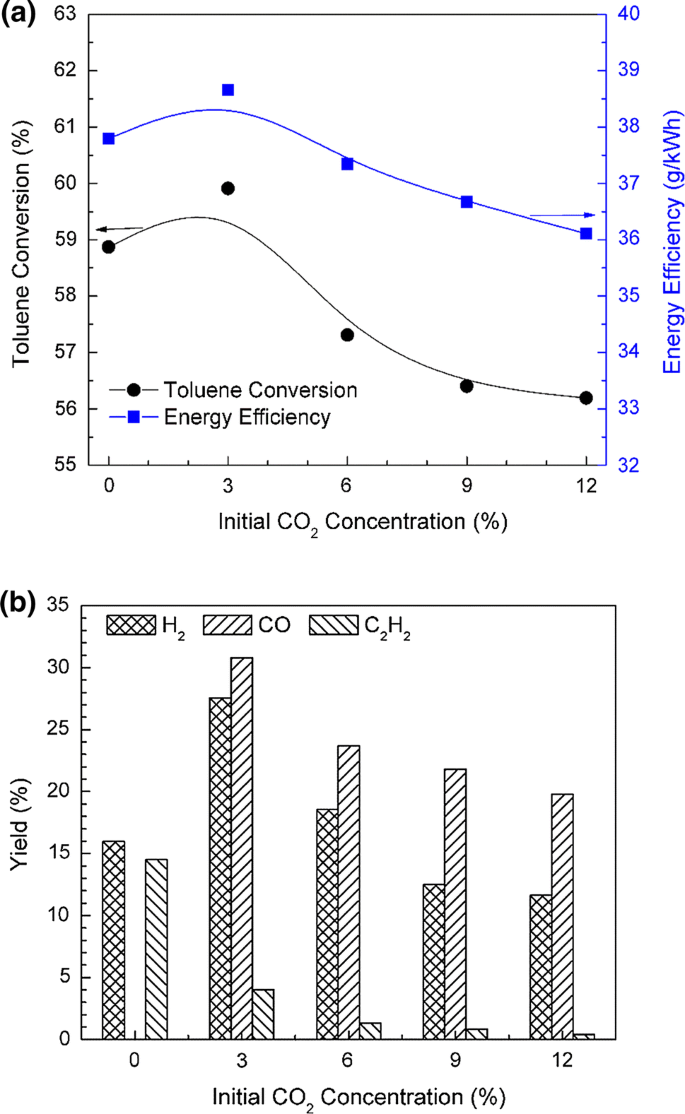Fig. 2
$${\text{e + N}}_{ 2} \to {\text{N}}_{ 2}^{ *} {\text{ + e}}$$
(R1)
$${\text{C}}_{ 6} {\text{H}}_{ 5} \cdot {\text{CH}}_{ 3} {\text{ + N}}_{ 2}^{ *} \to {\text{C}}_{ 6} {\text{H}}_{ 5} \cdot {\text{CH}}_{ 2} {\text{ + H + N}}_{ 2}$$
(R2)
$${\text{C}}_{ 6} {\text{H}}_{ 5} \cdot {\text{CH}}_{ 3} {\text{ + N}}_{ 2}^{ *} \to {\text{C}}_{ 6} {\text{H}}_{ 4} \cdot {\text{CH}}_{3} {\text{ + H + N}}_{ 2}$$
(R3)
$${\text{C}}_{ 6} {\text{H}}_{ 5} \cdot {\text{CH}}_{ 3} {\text{ + N}}_{ 2}^{ *} \to {\text{C}}_{ 6} {\text{H}}_{5} {\text{ + CH}}_{ 3} {\text{ + N}}_{ 2}$$
(R4)
$${\text{ C}}_{ 6} {\text{H}}_{ 5} \cdot {\text{CH}}_{3} {\text{ + N}}_{2}^{*} \to {\text{C}}_{ 5} {\text{H}}_{ 6} {\text{ + C}}_{ 2} {\text{H}}_{ 2} {\text{ + N}}_{ 2} ,$$
(R5)

where N2* can be either nitrogen metastable states N2 (A3∑) or nitrogen-excited states N2 (a′) and N2 (B). Adding CO2 to the plasma tar reforming reaction generates active oxygen species (e.g., O radicals, R6 and R7), which creates new reaction routes for the conversion of toluene. However, the presence of CO2 in the plasma tar reforming consumes energetic electrons (e.g., R6) and quenches the formed nitrogen-excited species (e.g., R7), resulted in a negative effect on the conversion of toluene and energy efficiency, especially at a higher CO2 concentration. Thus, the effect of CO2 concentration on the conversion of toluene and energy efficiency in the plasma tar reforming process is strongly dependent on the balance between these two opposite effects. In this work, the optimal CO2 content of 3 vol% was found to achieve the highest toluene conversion and energy efficiency:

$${\text{ CO}}_{ 2} {\text{ + e}} \to {\text{CO + O + e}}$$
(R6)
$${\text{ CO}}_{ 2} {\text{ + N}}_{2}^{*} \to {\text{CO + O + N}}_{ 2} .$$
(R7)

In addition, the presence of CO2 in the plasma reforming of toluene affected the distribution of gaseous products. H2 and C2H2 were identified as the major gas products in the plasma reaction without CO2. Hydrogen can be generated through the recombination of two H atoms, which are more likely produced by dehydrogenation of methyl group as the dissociation energy of C–H bond (3.7 eV) in methyl group is the weakest in toluene [26, 32]. Acetylene is mainly produced via the cleavage of benzene rings by nitrogen-excited species (R5). However, increasing CO2 content from 0% to 12 vol% significantly decreased the yield of C2H2 by a factor of 37. In contrast, the formation of CO was observed in the presence of CO2. The yield of CO and hydrogen reached a peak at the optimal CO2 content of 3 vol% and then decreased when further increasing CO2 content to 12 vol%, which was strongly related to the change of toluene conversion as a function of CO2 content.

To better understand the role of CO2 in the plasma reforming of toluene, optical emission spectroscopic diagnostics was used to investigate the formation of different reactive species in the plasma process. Figure 3 plots the emission spectra of N2/C7H8 and N2/CO2/C7H8 plasmas in the spectral range of 200–600 nm. Clearly, the spectrum of N2/C7H8 GAD is dominated by the CN ($$B^{2} \sum \to X^{2} \sum$$) violet system and C2 swan bands.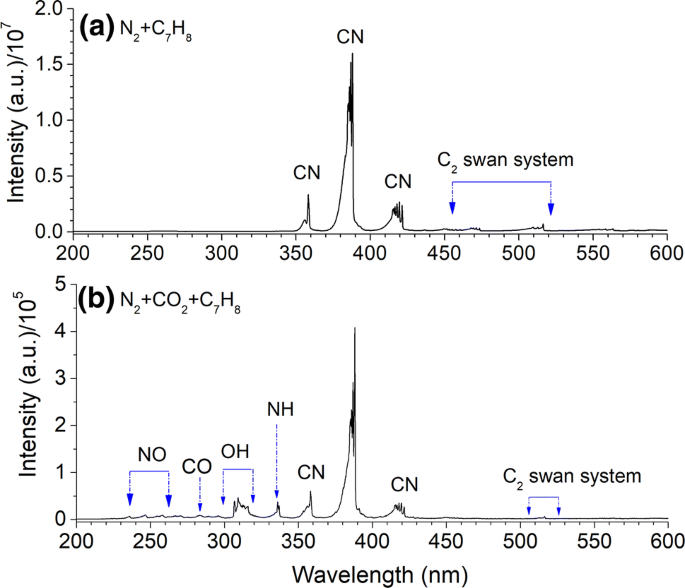Fig. 3

The presence of CO2 in the plasma reforming of toluene produces new chemically reactive species. For instance, OH ($$A^{2} \sum^{ + } \to X^{2} \prod$$) bands can be observed in the spectrum of the N2/C7H8/CO2 GAD. The formation of OH radicals in the plasma CO2 reforming of toluene can be attributed to the reactions of CO2 (or O and CO) with toluene and its fragments. The introduction of CO2 to the reforming of toluene reduces the formation of carbon deposition, which can also be demonstrated by the reduced relative intensity of CN bands (Fig. 3). This finding also reveals that the formation of CN bands in the OES of N2/C7H8 and N2/CO2/C7H8 plasmas can be associated with carbon deposition in the plasma reforming of toluene (R8 and R9):

$${\text{N}}_{2} + {\text{C}} \to {\text{CN}} + {\text{N}}$$
(R8)
$${\text{N}}_{2} + {\text{C}} + {\text{N}} \to {\text{CN}} + {\text{N}}_{2} .$$
(R9)

The spectrum of the N2/C7H8/CO2 GAD shows an NH ($$A^{2} \sum \to X^{2} \sum$$) band at 336.1 nm (Fig. 3 and Fig. 4), while this band cannot be observed in the spectrum of the N2/C7H8 GAD, which suggests that the formation of NH might be related to the reaction of excited nitrogen species with OH radicals . This phenomenon also reveals that the formation of NH via direct reactions between N2 and H2 might be negligible.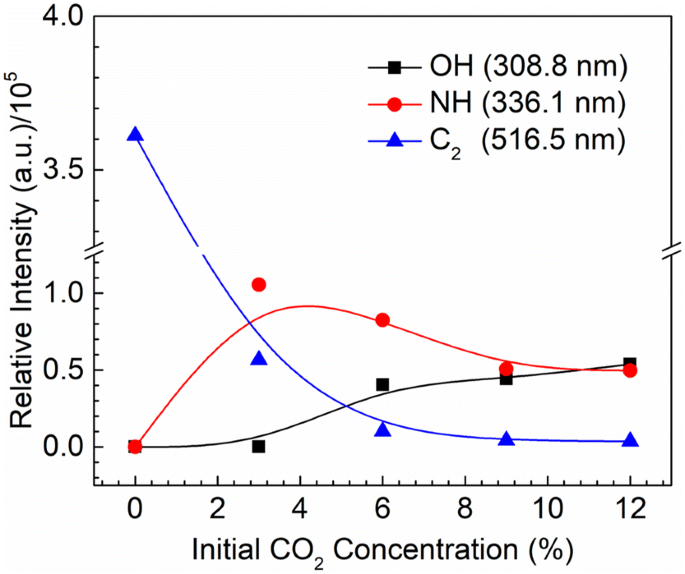Fig. 4

### Effect of S/C molar ratio

Figure 5a plots the effect of S/C molar ratio on the conversion of toluene and energy efficiency in the plasma CO2 reforming of toluene. The concentration of CO2 in producer gas from biomass gasification is in the range of 10–13 vol%, and therefore, the volume percentage of CO2 in the plasma reforming of toluene was fixed at 12 vol%. The highest toluene conversion (63.3%) and energy efficiency (40.5 g/kWh) were achieved at the optimum S/C molar ratio of 1.0.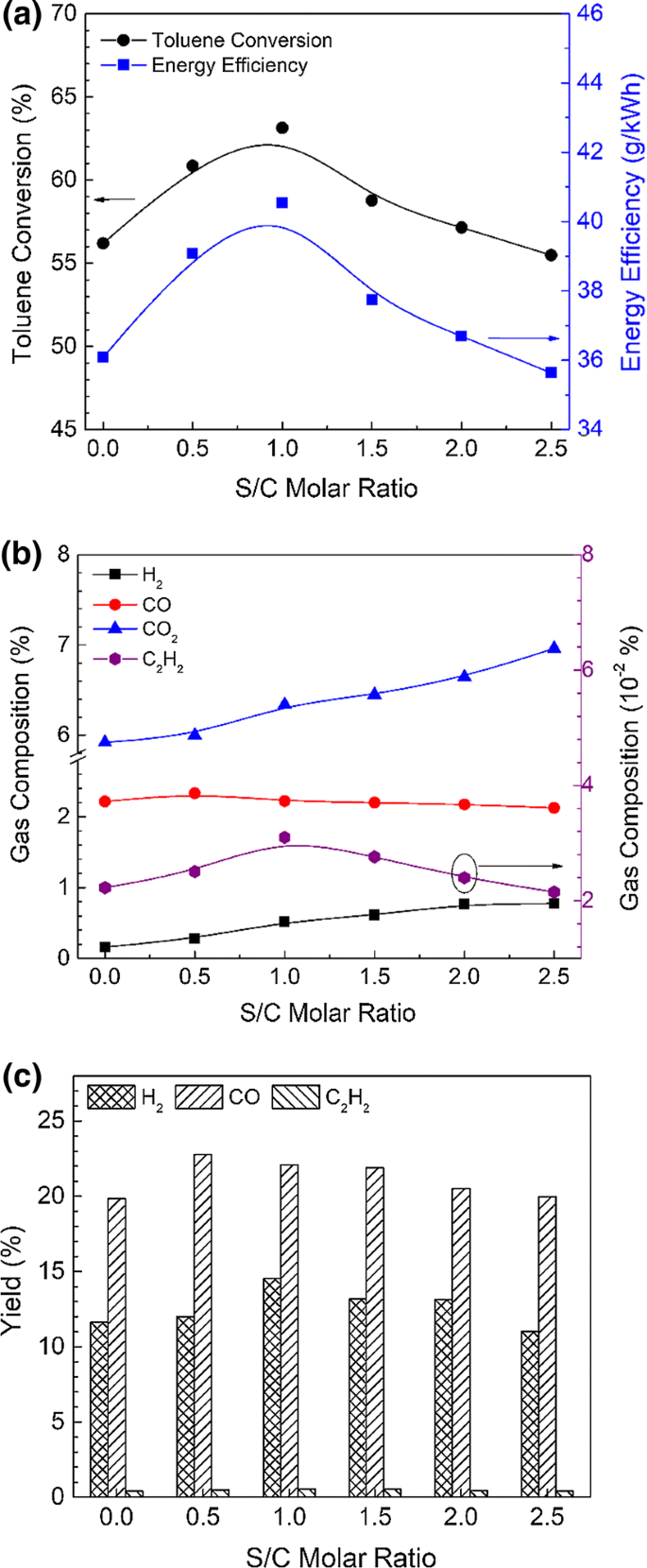Fig. 5

The presence of steam in the plasma reforming of toluene generates OH radicals via H2O dissociation by energetic electrons (R10) and nitrogen-excited species such as N2 (A3Σ+) (R11), creating new reaction pathways for the oxidation of toluene and reaction intermediates. It is well recognized that OH radicals are much more reactive in oxidation reactions compared to atomic oxygen at low temperatures. The reaction rate of R12 is about two orders of magnitude higher than that of R13 . However, further increasing S/C molar ratio from 1.0 to 2.5 substantially reduced the conversion of toluene. Due to the electronegative character of H2O, electron attachment of H2O molecules (R14) could take place and consequently reduces the available electrons and metastable nitrogen species for toluene decomposition. Thus, the effect of steam on the conversion of toluene is strongly dependent on the balance between these two opposite effects: (1) enhanced toluene conversion via additional oxidation routes due to the positive effect of OH radicals; and (2) reduced toluene conversion resulted from the negative effect of electron attachment on water and reduced available electrons and nitrogen-excited species:

$${\text{H}}_{ 2} {\text{O}} + {\text{e}} \to {\text{H}} + {\text{OH}} + {\text{e}}$$
(R10)
$${\text{H}}_{2} {\text{O}} + {\text{N}}_{2} \left( {A^{3}\Sigma ^{ + } } \right) \to {\text{H}} + {\text{OH}} + {\text{N}}_{2}$$
(R11)
$${\text{ C}}_{6} {\text{H}}_{5} \cdot {\text{CH}}_{ 3} {\text{ + OH }} \to {\text{C}}_{ 6} {\text{H}}_{ 5} \cdot {\text{CH}}_{ 2} {\text{ + H}}_{ 2} {\text{O}}$$
(R12)
\begin{aligned} {\text{C}}_{ 6} {\text{H}}_{ 5} \cdot {\text{CH}}_{ 2} \;{ + }\;{\text{O}} & \to {\text{C}}_{ 6} {\text{H}}_{5} \cdot {\text{CHO}}\;{ + }\;{\text{OH}} \\ & \to {\text{C}}_{ 6} {\text{H}}_{5} \cdot {\text{CO}}\;{ + }\;{\text{H}}_{ 2} {\text{O}} \\ \end{aligned}
(R13)
$${\text{H}}_{ 2} {\text{O}} + {\text{e}} \to {\text{H}}_{2} {\text{O}}^{ - } .$$
(R14)

In addition, changing S/C molar ratio also changes the distribution of gas products. As shown in Fig. 5, increasing the S/C molar ratio from 0 to 2.5 increases the volume fraction of H2 and CO2 in the gaseous products, whereas the concentration of CO gradually decreases with increasing the S/C molar ratio. This phenomenon can be attributed to the occurrence of the water–gas shift reaction (R15). In contrast, the evolution of C2H2 concentration with the S/C molar ratio is very similar to the effect of S/C ratio on the toluene conversion:

$${\text{H}}_{ 2} {\text{O}} + {\text{CO}} \to {\text{H}}_{2} + {\text{CO}}_{2} .$$
(R15)

### Reaction pathways and mechanism

Table 1 summarizes the formation of organic by-products in the plasma reforming of toluene under different conditions. The presence of CO2 or CO2/H2O in the plasma toluene reforming reduced the production of by-products due to the enhanced oxidation of toluene and its fragments. Benzonitrile and 1-isocyano-4-methylbenzene could be formed through the recombination of CN radicals with phenyl and benzyl, respectively. Both products were found in the plasma reforming of toluene, but cannot be observed when introducing CO2 or steam to the toluene reforming. The detected by-products listed in Table 1 can be divided into three major groups. The first group of the by-products contains one-ringed compounds, such as styrene, ethylbenzene, and phenylethyne, generated through alkylation of benzene with ethylene, ethane, or ethyne, respectively. The oxygen-containing substituents’ monocyclic aromatic compounds such as 4-aminobenzoic acid and benzeneacetamide were also observed, indicating that the reactions between intermediates and nitrogen oxides take place in the plasma tar reforming. This finding also reveals that N, O, and hydroxyl radicals do not directly react with toluene, but are consumed to produce carboxyl acid, hydroxylamine (–N–OH), and carboxamide (–CO–NR) compounds. Moreover, naphthalene and bibenzyl belong to the second group (polycyclic hydrocarbons), which reveals the recombination of toluene fragments takes place and forms large molecular polymer compounds. Note that no products with more than two aromatic rings were found in this experiment. Finally, linear organic by-products represent the third group including 1, 3-butadiyne and oxalic acid, which could be produced through the cleavage of toluene followed by hydrogenation and oxidation. Remarkably, the presence of aliphatic compound (dodecane) implies that C2 and C3 entities tend to polymerize to form linear hydrocarbons, in a kerosene-to-light diesel oil range.

Plasma reforming of toluene is a complex process involving a range of physical and chemical reactions. Concerning the small electron cross section of aromatic hydrocarbons (e.g., toluene), the direct electron impact dissociation has a weak contribution to the destruction of toluene , while the dissociation of toluene by nitrogen-excited species plays a more critical role in the decomposition of toluene . In the presence of steam, oxidation of toluene and its fragments by OH radicals has also been considered as an important reaction route for toluene conversion. Figure 6 shows plausible reaction pathways in the plasma reforming of toluene with steam and CO2.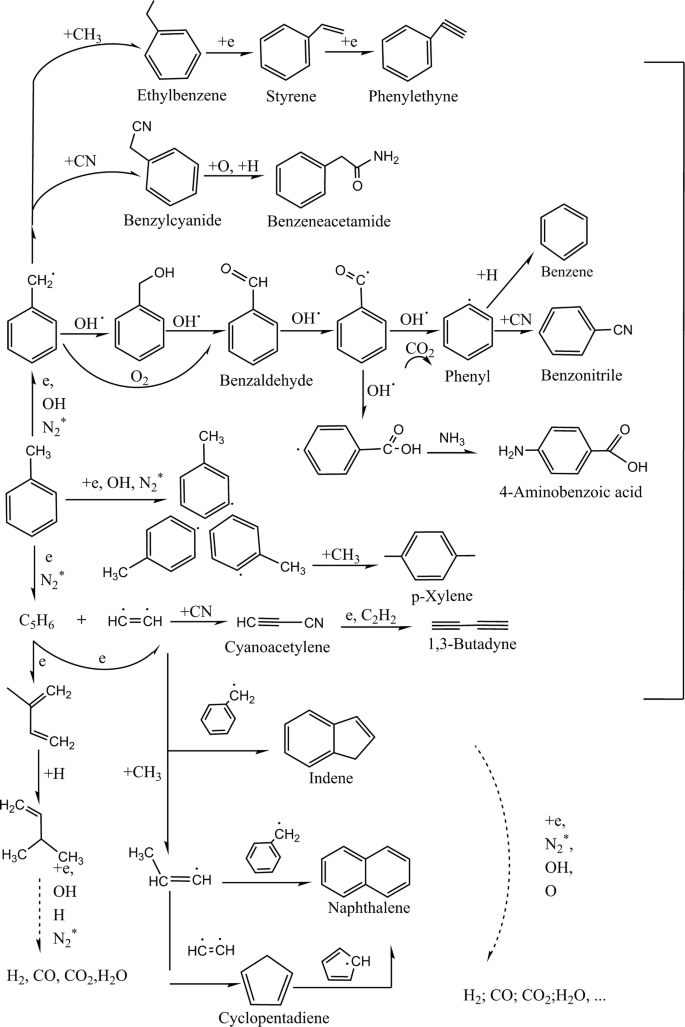Fig. 6

## Conclusions

In this paper, the influence of CO2 and steam on the reforming of toluene has been investigated in a gliding arc discharge reactor. We find that both CO2 and steam significantly affect the conversion of toluene and the production of gas and liquid products. Hydrogen and C2H2 were identified as the major gas products in the plasma reforming of toluene without CO2 or steam, while syngas was found as the primary products with a maximum yield of 58.3% (27.5% for H2 and 30.8% for CO) in the plasma reforming with the addition of 12 vol% CO2. The results show that the optimal CO2 content was 3 vol% to achieve the highest toluene conversion of 59.9% in the plasma reforming of toluene without adding steam, while the maximum conversion of toluene (63.3%) and energy efficiency (40.5 g/kWh) in the plasma CO2 reforming of toluene was achieved at an S/C molar ratio of 1.0. The presence of CO2 and steam in the plasma reforming creates extra reactive species and reaction pathways which contribute to the varied conversion of toluene and energy efficiency. In addition, the produced oxidative species in the plasma reforming of toluene contributed to the reduced formation of condensable by-products. The plausible reaction pathways have been proposed and discussed through a comprehensive analysis of gaseous and liquid products combined with OES diagnostics.

## References

1. 1.

Kong M, Yang Q, Fei J, Zheng X. Experimental study of Ni/MgO catalyst in carbon dioxide reforming of toluene, a model compound of tar from biomass gasification. Int J Hydrog Energy. 2012;37:13355–64.

2. 2.

Warsita A, Al-attab KA, Zainal ZA. Effect of water addition in a microwave assisted thermal cracking of biomass tar models. Appl Therm Eng. 2017;113:722–30.

3. 3.

Milne TA, Evans RJ, Abatzoglou N. Biomass gasifier “tars”: their nature, formation, and conversion. Lakewood: National Renewable Energy Laboratory; 1998.

4. 4.

Tao K, Ohta N, Liu GQ, Yoneyama Y, Wang T, Tsubaki N. Plasma enhanced catalytic reforming of biomass tar model compound to syngas. Fuel. 2013;104:53–7.

5. 5.

Shen Y, Yoshikawa K. Recent progresses in catalytic tar elimination during biomass gasification or pyrolysis—a review. Renew Sust Energy Rev. 2013;21:371–92.

6. 6.

Bhave AG, Vyas DK, Patel JB. A wet packed bed scrubber-based producer gas cooling-cleaning system. Renew Energy. 2008;33:1716–20.

7. 7.

Fagbemi L, Khezami L, Capart R. Pyrolysis products from different biomasses: application to the thermal cracking of tar. Appl Energy. 2001;69:293–306.

8. 8.

Chen T, Liu H, Shi P, Chen D, Song L, He H, Frost RL. CO2 reforming of toluene as model compound of biomass tar on Ni/palygorskite. Fuel. 2013;107:699–705.

9. 9.

Saleem F, Zhang K, Harvey A. Role of CO2 in the conversion of toluene as a tar surrogate in a nonthermal plasma dielectric barrier discharge reactor. Energy Fuel. 2018;32:5164–70.

10. 10.

Liu L, Zhang Z, Das S, Kawi S. Reforming of tar from biomass gasification in a hybrid catalysis-plasma system: a review. Appl Catal B: Environ. 2019;250:250–72.

11. 11.

Wnukowski M, Jamróz P. Microwave plasma treatment of simulated biomass syngas: interactions between the permanent syngas compounds and their influence on the model tar compound conversion. Fuel Process Technol. 2018;173:229–42.

12. 12.

Xu B, Xie J, Zhan H, Yin X, Wu C, Liu H. Removal of toluene as a biomass tar surrogate in a catalytic nonthermal plasma process. Energy Fuel. 2018;32:10709–19.

13. 13.

Liu S, Mei D, Wang L, Tu X. Steam reforming of toluene as biomass tar model compound in a gliding arc discharge reactor. Chem Eng J. 2017;307:793–802.

14. 14.

Mei D, Liu S, Tu X. CO2 reforming with methane for syngas production using a dielectric barrier discharge plasma coupled with Ni/γ-Al2O3 catalysts: process optimization through response surface methodology. J CO2 Util. 2017;21:314–26.

15. 15.

Ashford B, Tu X. Non-thermal plasma technology for the conversion of CO2. Curr Opin Green Sust Chem. 2017;3:45–9.

16. 16.

Van Durme J, Dewulf J, Sysmans W, Leys C, van Langenhove H. Abatement and degradation pathways of toluene in indoor air by positive corona discharge. Chemosphere. 2007;68:1821–9.

17. 17.

Nair SA, Pemen AJM, Yan K, van Gompel FM, van Leuken HEM, van Heesch EJM, Ptasinski KJ, Drinkenburg AAH. Tar removal from biomass-derived fuel gas by pulsed corona discharges. Fuel Process Technol. 2003;84:161–73.

18. 18.

Ni M-J, Shen X, Gao X, Wu Z-L, Lu H, Li Z-S, Luo Z-Y, Cen K-F. Naphthalene decomposition in a DC corona radical shower discharge. J Zhejiang Univ-Sci A. 2011;12:71–7.

19. 19.

Blin-Simiand N, Jorand F, Magne L, Pasquiers S, Postel C, Vacher JR. Plasma reactivity and plasma-surface interactions during treatment of toluene by a dielectric barrier discharge. Plasma Chem Plasma Process. 2008;28:429–66.

20. 20.

Abdelaziz AA, Seto T, Abdel-Salam M, Otani Y. Performance of a surface dielectric barrier discharge based reactor for destruction of naphthalene in an air stream. J Phys D Appl Phys. 2012;45:115201.

21. 21.

Chang C-L, Bai H, Lu S-J. Destruction of styrene in an air stream by packed dielectric barrier discharge reactors. Plasma Chem Plasma Process. 2005;25:641–57.

22. 22.

Lee B, Kim D-W, Park D-W. Dielectric barrier discharge reactor with the segmented electrodes for decomposition of toluene adsorbed on bare-zeolite. Chem Eng J. 2019;357:188–97.

23. 23.

Jamróz P, Kordylewski W, Wnukowski M. Microwave plasma application in decomposition and steam reforming of model tar compounds. Fuel Process Technol. 2018;169:1–14.

24. 24.

Sekine Y, Urasaki K, Kado S, Matsukata M, Kikuchi E. Nonequilibrium pulsed discharge: a novel method for steam reforming of hydrocarbons or alcohols. Energy Fuel. 2004;18:455–9.

25. 25.

Nunnally T, Tsangaris A, Rabinovich A, Nirenberg G, Chernets I, Fridman A. Gliding arc plasma oxidative steam reforming of a simulated syngas containing naphthalene and toluene. Int J Hydrog Energy. 2014;39:11976–89.

26. 26.

Zhu FS, Li XD, Zhang H, Wu AJ, Yan JH, Ni MJ, Zhang HW, Buekens A. Destruction of toluene by rotating gliding arc discharge. Fuel. 2016;176:78–85.

27. 27.

Yang YC, Chun YN. Naphthalene destruction performance from tar model compound using a gliding arc plasma reformer. Korean J Chem Eng. 2011;28:539–43.

28. 28.

Zhang H, Zhu F, Li X, Xu R, Li L, Yan J, Tu X. Steam reforming of toluene and naphthalene as tar surrogate in a gliding arc discharge reactor. J Hazard Mater. 2019;369:244–53.

29. 29.

Wang Y, Yang H, Tu X. Plasma reforming of naphthalene as a tar model compound of biomass gasification. Energy Convers Manag. 2019;187:593–604.

30. 30.

Tu X, Whitehead JC. Plasma dry reforming of methane in an atmospheric pressure AC gliding arc discharge: co-generation of syngas and carbon nanomaterials. Int J Hydrog Energy. 2014;39:9658–69.

31. 31.

Mei D, Wang Y, Liu S, Alliati M, Yang H, Tu X. Plasma reforming of biomass gasification tars using mixed naphthalene and toluene as model compounds. Energy Convers Manag. 2019;195:409–19.

32. 32.

Liu SY, Mei DH, Nahil MA, Gadkari S, Gu S, Williams PT, Tu X. Hybrid plasma-catalytic steam reforming of toluene as a biomass tar model compound over Ni/Al2O3 catalysts. Fuel Process Technol. 2017;166:269–75.

33. 33.

Zhang H, Zhu F, Li X, Cen K, Du C, Tu X. Rotating gliding arc assisted water splitting in atmospheric nitrogen. Plasma Chem Plasma Process. 2016;36:813–34.

34. 34.

Abdelaziz AA, Seto T, Abdel-Salam M, Otani Y. Influence of N2/O2 mixtures on decomposition of naphthalene in surface dielectric barrier discharge based reactor. Plasma Chem Plasma Process. 2014;34:1371–85.

35. 35.

Mei D, Liu S, Wang Y, Yang H, Bo Z, Tu X. Enhanced reforming of mixed biomass tar model compounds using a hybrid gliding arc plasma catalytic process. Catal Today. 2019. https://doi.org/10.1016/j.cattod.2019.05.046.

Download references

## Acknowledgements

The support of this work by the UK EPSRC Impact Acceleration Account (IAA) is gratefully acknowledged. This project has received funding from the European Union’s Horizon 2020 research and innovation program under the Marie Skłodowska-Curie Grant agreement No. 823745.

## Author information

Authors

### Corresponding author

Correspondence to Xin Tu.

## Additional information

### Publisher's Note

Springer Nature remains neutral with regard to jurisdictional claims in published maps and institutional affiliations.

## Rights and permissions

Open Access This article is distributed under the terms of the Creative Commons Attribution 4.0 International License (http://creativecommons.org/licenses/by/4.0/), which permits unrestricted use, distribution, and reproduction in any medium, provided you give appropriate credit to the original author(s) and the source, provide a link to the Creative Commons license, and indicate if changes were made.

Reprints and Permissions